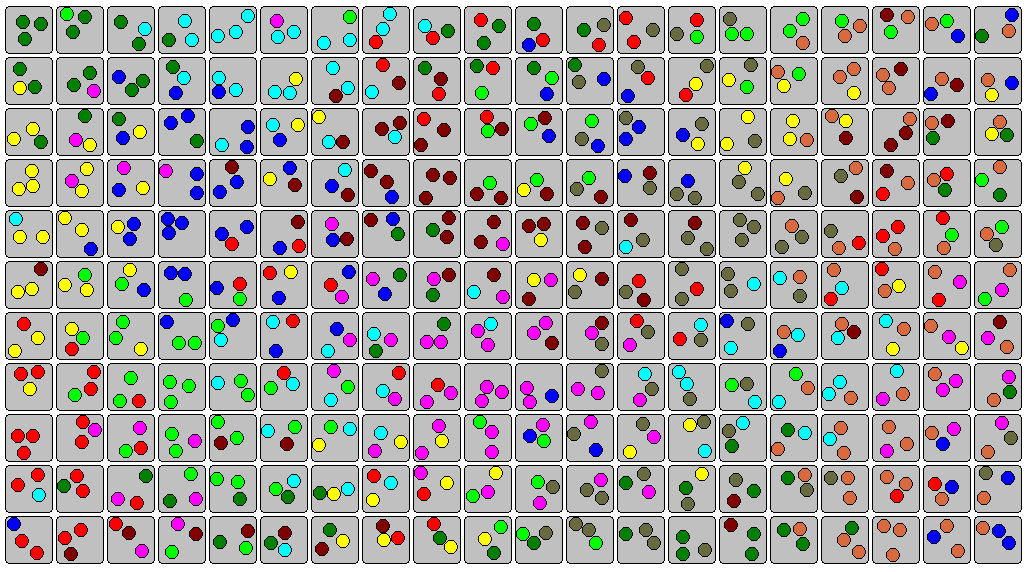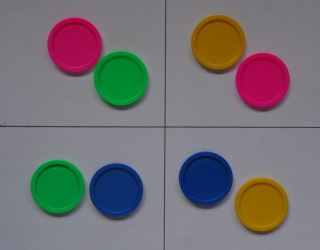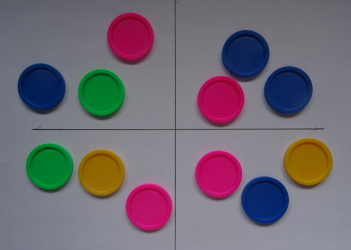# Matching Multisets

2019/09/04Let n be the number of items to choose from. If you take k elements without repetition you can get n C k different sets or combinations with n C k the binomial coefficients. If you take the elements with repetition allowed you can get (n+k-1) C k different multisets. Jared McComb asked to arrange the multisets as a rectangle so that all neighboring multisets only differ in one element.
The number of matching sides and therefore the difficulty to find a solution essentially depends on the side lengths of the rectangles. A (w x 1) rectangle looks like a chain and has only w-1 touching sides but a (a x b) rectangle with a*b=w has (a-1)b+a(b-1) of them. Therefore chains are easily to solve and almost squares are hard.

For chains of small multisets a systematic appraoch seem to be possible. If each piece has only two neighbors or less it's easy to meet the condition that neighboring pieces differ in only one element.

Let (a,b) with 1<=a<=b<=n a multiset of cardinalty 2 from n objects. We can start with a chain like this: (1,1) (1,2) (2,2) (2,3) (3,3)...(n-1,n-1) (n-1,n) (n,n) In this chain pieces (a,b) witb b>a+1 are missing. If a=1 they can be inserted between (1,1) and (1,2). If 2<=a<=n the pieces can be inserted between (a-1,a) and (a,a), This way all pieces are placed.

Let (a,b,c) with 1<=a<=b<=c<=n a multiset of cardinality 3 from n objects. For a chain of these pieces a systematic approach is also possible. First we take all pieces with three or two equal elements. With these pieces we can get a chain like this: (1,1,1)(1,1,n)(1,1,n-1)...(1,1,2)(1,2,2)(2,2,2)(2,2,n)...(2,2,3)(2,3,3)(3,3,3).... ...(n-2,n-2,n-2)(n-2,n-2,n)(n-2,n-2,n-1)(n-2,n-1,n-1)(n-1,n-1,n-1)(n-1,n-1,n)(n-1,n,n)(n,n,n) For each a>1 just before (a,a,a) the piece (a-1,a,a) is inserted and all other pieces with two "a-1" are placed in descending order between (a-1,a-1,a-1) and (a-1,a,a). What about the pieces (a,b,b+1)...(a,b,n) with three different elements. They can be inserted between (a,a,b+1) and (a,a,b) in the chain above. Because of the chosen descending order in each section such a pair of neighboring pieces is always available.

For further rectangles I wrote a program to find solutions by backtracking. Click the pictures to see solutions for multisets of cardinality from 2 up to 4. For "multisets" with only one element the matching condition if fulfilled by definition.Beside the rectangles you can construct spirals of width 1, 2 and 3. The space to display such structures is much smaller than the space needed for long rectangles.Home